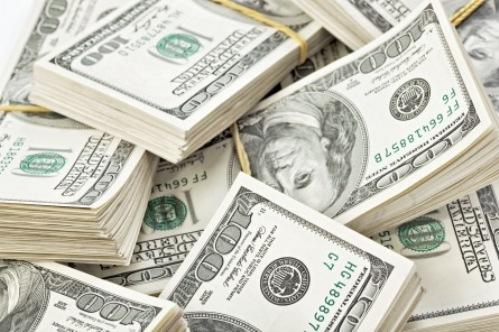UFC 200 - Les salaires
Publié le : Mardi 12 juillet 2016Brock Lesnar encaisse 2 500 000 \$, Mark Hunt encaisse 700 000 \$....

Voir la carte de l'UFC 200

Amanda Nunes (\$100,000)
Miesha Tate (\$500,000)
Brock Lesnar (\$2,500,000)
Mark Hunt (\$700,000)
Daniel Cormier (\$500,000)
Anderson Silva (\$600,000)
Jose Aldo (\$400,000 + \$100,000 = \$500,000)
Frankie Edgar (\$190,000)
Cain Velasquez (\$300,000)
Travis Browne (\$120,000)
Julianna Pena (\$32,000 + \$32,000 = \$64,000)
Cat Zingano (\$35,000)
Kelvin Gastelum (\$53,000 + \$33,000 = \$83,000)
Johny Hendricks (\$80,000)
T.J. Dillashaw (\$25,000 + \$25,000 = \$50,000)
Raphael Assuncao (\$42,000)
Sage Northcutt (\$50,000 + \$50,000 = \$100,000)
Enrique Marin (\$13,000)
Joe Lauzon (\$54,000 + \$54,000 = \$108,000)
Diego Sanchez (\$80,000)
Gegard Mousasi (\$75,000 + \$35,000 = \$110,000)
Thiago Santos (\$28,000)
Jim Miller (\$59,000 + \$59,000 = \$118,000)
Takanori Gomi (\$55,000)

Retour